# Scatterplot Jittering

Scatterplots are one of the best ways to understand a bivariate relationship. They neatly show the form of the relationship between x and y. But they are really only effective when both variables are continuous. When one of the variables in discrete, boxplots, conditional density plots, and other visualization techniques often do a better job communicating relationships. But sometimes we have discrete data that is almost continuous (e.g., years of formal education). These kinds of variables might be nearly continuous and have approximately linear relationships with other variables. Summarizing an continuous outcome (e.g., income) using a boxplot at every level of education can be pretty tedious and indeed is a difficult graph to read. In these situations, we might want to rely on a scatterplot, but we need to preprocess the data in order to clearly visualize it.

Let's start with some example data (where the predictor variable is discrete and the outcome is continuous), look at the problems with plotting these kinds of data using R's defaults, and then look at the jitter function to draw a better scatterplot.

set.seed(1)
x <- sample(1:10, 200, TRUE)
y <- 3 * x + rnorm(200, 0, 5)


Here's what a standard scatterplot of these data looks like:

plot(y ~ x, pch = 15)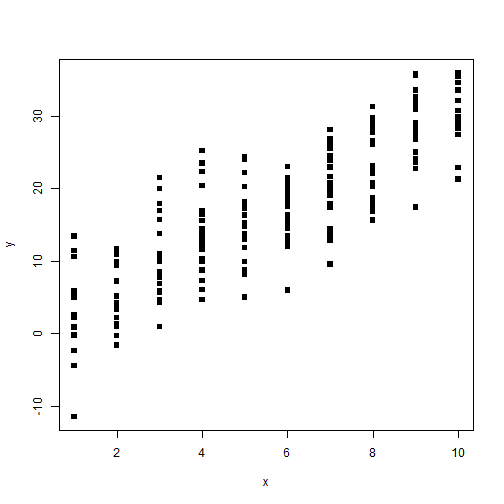Because the independent variable is only observed at a few levels, it can be difficult to get a sense of the “cloud” of points. We can use jitter to add a little random noise to the data in order to see the cloud more clearly:

plot(y ~ jitter(x, 1), pch = 15)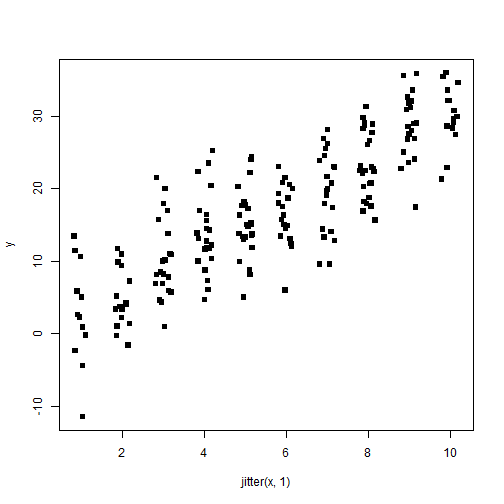We can add even more random noise to see an even more “cloud”-like representation:

plot(y ~ jitter(x, 2), pch = 15)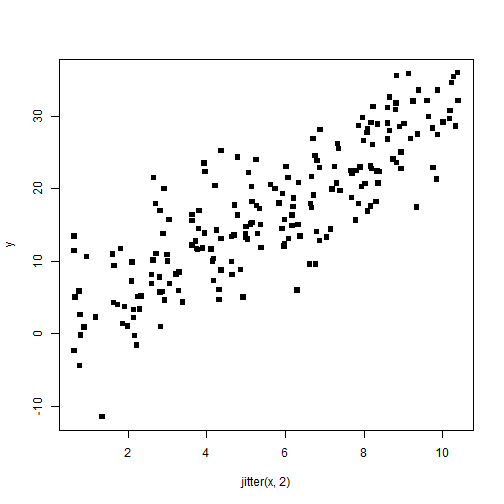If both our independent and dependent variables are discrete, the value of jitter is even greater. Let's look at some data like this:

x2 <- sample(1:10, 500, TRUE)
y2 <- sample(1:5, 500, TRUE)
plot(y2 ~ x2, pch = 15)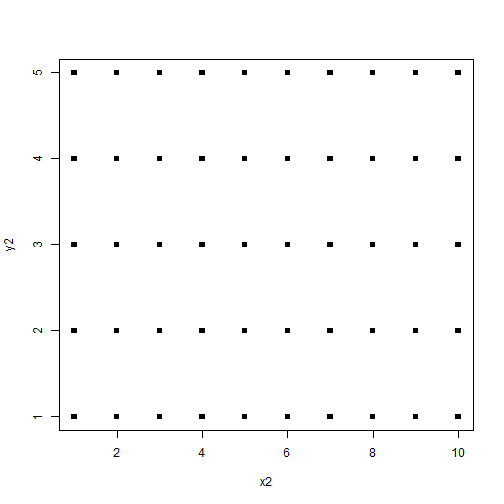Here the data simply look like a grid of points. It is impossible to infer the density of the data anywhere in the plot. jitter will be quite useful. Let's start by applying jitter just to the x2 variable (as we did above):

plot(y2 ~ jitter(x2), pch = 15)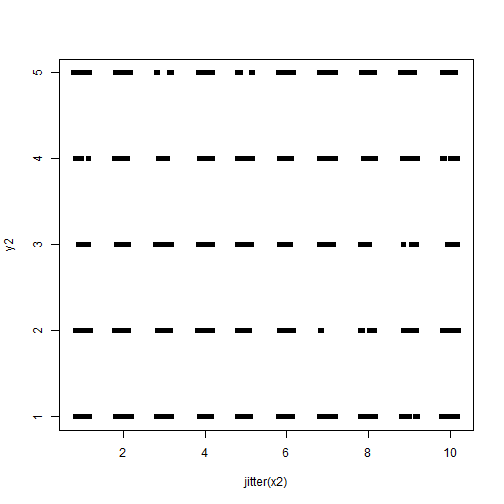Here we start to see teh data a little more clearly. Let's try it just on the outcome:

plot(jitter(y2) ~ x2, pch = 15)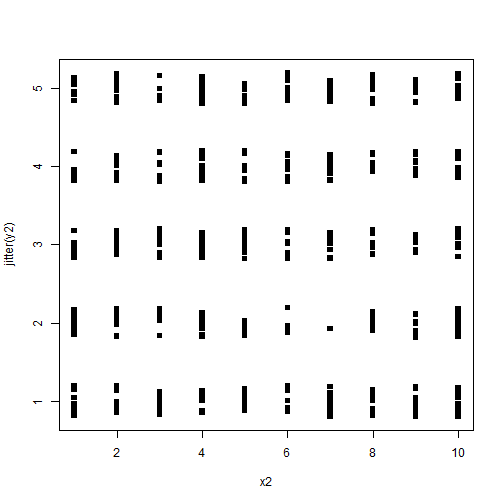That's a similar level of improvement, but let's use jitter on both the outcome and predictor to get a much more cloud-like effect:

plot(jitter(y2) ~ jitter(x2), pch = 15)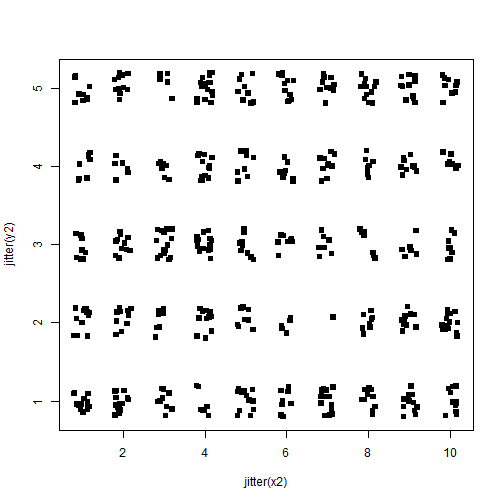Adding even more noise will make an even fuller cloud:

plot(jitter(y2, 2) ~ jitter(x2, 2), pch = 15)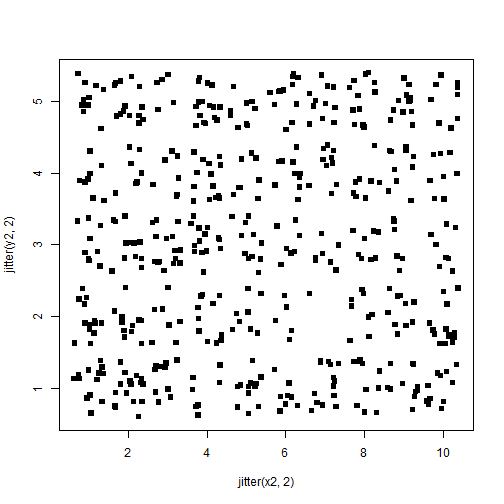We now clearly see that our data are evenly dense across the entire matrix. Of course, adding this kind of noise probably isn't appropriate for analyzing data, but we could, e.g., run a regression model on the original data then when we plot the results use the jitter inputs in order to more clearly convey the underlying descriptive relationship.